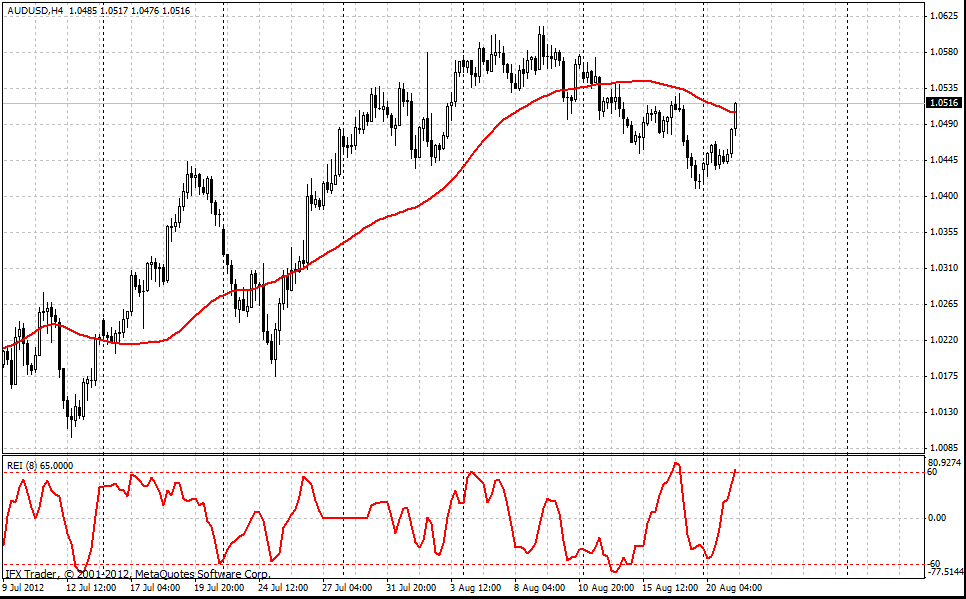# 范围扩展指数

#### 公式

S1 = Sum(k, j=1) n(j)m(j)s(j)

if ((High[j - 2] < Close[j - 7]) && (High[j - 2] < Close[j - 8]) && (High[j] < High[j - 5]) && (High[j] < High[j - 6])) n(j) = 0, else n (j) = 1

m(j)是第二种情况:

if ((Low[j - 2] > Close[j - 7]) && (Low[j - 2] > Close[j - 8]) && (Low[j] > Low[j - 5]) && (Low[j] > Low[j - 6])) m(j) = 0, else m(j) = 1

s(j) = High[j]-High[j-2]+Low[j] - Low[j-2]

S(2) = Sum(k, j=1) |High[j]-High[j-2]|+|Low[j] - Low[j-2]|

REI = S1/S2*100

#### 交易应用

- 如果REI产生极端+60以上的水平同时价格低于均线（50），短期建仓。
- 如果REI产生极端-60以下的水平同时价格高于均线（50），长期建仓。#### InstaForex REI 参数

REI_period = 8